# 1.基本概念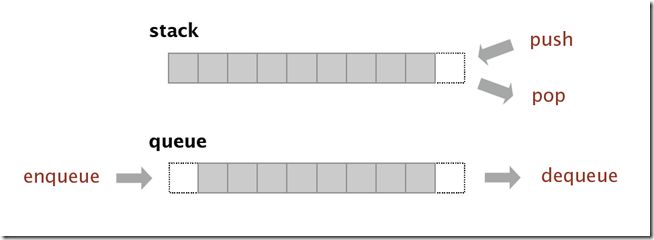# 2.实现

1.1 Stack的实现Stack() 创建一个空的栈
void Push(T s) 往栈中添加一个新的元素
T Pop() 移除并返回最近添加的元素
boolean IsEmpty() 栈是否为空
int Size() 栈中元素的个数

``````class Node
{
public T Item{get;set;}
public Node Next { get; set; }
}
private Node first = null;
private int number = 0;``````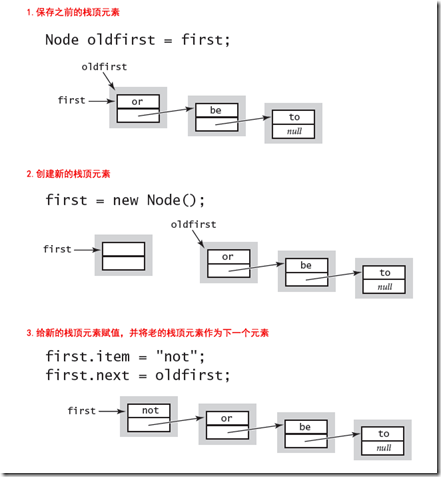``````void Push(T node)
{
Node oldFirst = first;
first = new Node();
first.Item= node;
first.Next = oldFirst;
number++;
}``````

Pop方法也很简单，首先保存栈顶元素的值，然后将栈顶元素设置为下一个元素：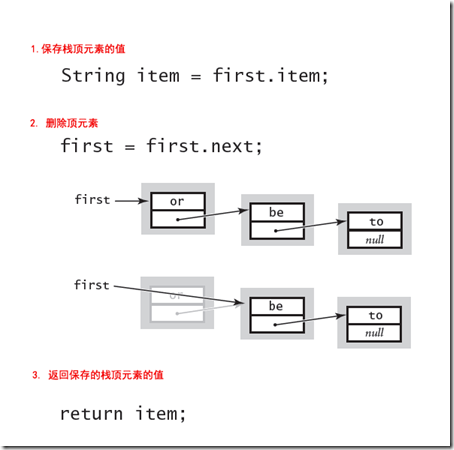``````T Pop()
{
T item = first.Item;
first = first.Next;
number--;
return item;
}``````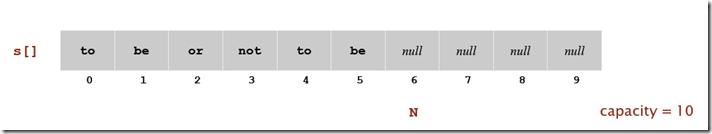``````T[] item;
int number = 0;

public StackImplementByArray(int capacity)
{
item = new T[capacity];
}
public void Push(T _item)
{
if (number == item.Length) Resize(2 * item.Length);
item[number++] = _item;
}``````

Pop方法：

``````public T Pop()
{
T temp = item[--number];
item[number] = default(T);
if (number > 0 && number == item.Length / 4) Resize(item.Length / 2);
return temp;
}``````

Resize方法基本就是数组复制：

``````private void Resize(int capacity)
{
T[] temp = new T[capacity];
for (int i = 0; i < item.Length; i++)
{
temp[i] = item[i];
}
item = temp;
}``````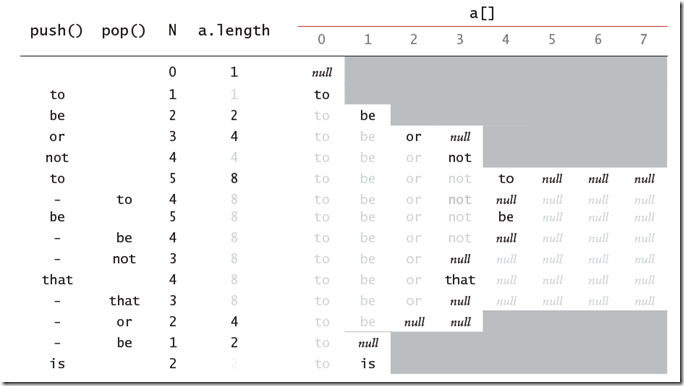2.2 Queue的实现

Queue是一种先进先出的数据结构，和Stack一样，他也有链表和数组两种实现，理解了Stack的实现后，Queue的实现就比较简单了。Stack() 创建一个空的队列
void Enqueue(T s) 往队列中添加一个新的元素
T Dequeue() 移除队列中最早添加的元素
boolean IsEmpty() 队列是否为空
int Size() 队列中元素的个数

Dequeue方法就是返回链表中的第一个元素，这个和Stack中的Pop方法相似：

``````public T Dequeue()
{
T temp = first.Item;
first = first.Next;
number--;
if (IsEmpety())
last = null;
return temp;
}``````

Enqueue和Stack的Push方法不同，他是在链表的末尾增加新的元素：

``````public void Enqueue(T item)
{
Node oldLast = last;
last = new Node();
last.Item = item;
if (IsEmpety())
{
first = last;
}
else
{
oldLast.Next = last;
}
number++;
}``````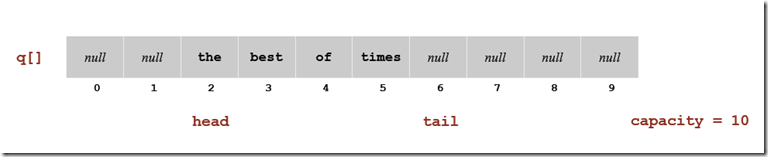``````public void Enqueue(T _item)
{
if ((head - tail + 1) == item.Length) Resize(2 * item.Length);
item[tail++] = _item;
}

public T Dequeue()
{
if (head > 0 && (tail - head + 1) == item.Length / 4) Resize(item.Length / 2);
return temp;
}

private void Resize(int capacity)
{
T[] temp = new T[capacity];
int index = 0;
for (int i = head; i < tail; i++)
{
temp[++index] = item[i];
}
item = temp;
}``````

# 3. .NET中的Stack和Queue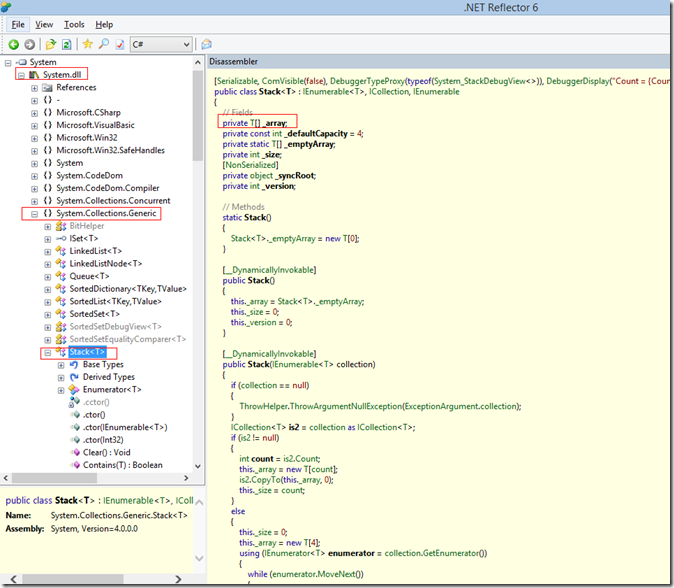``````[Serializable, ComVisible(false), DebuggerTypeProxy(typeof(System_StackDebugView<>)), DebuggerDisplay("Count = {Count}"), __DynamicallyInvokable]
public class Stack<T> : IEnumerable<T>, ICollection, IEnumerable
{
// Fields
private T[] _array;
private const int _defaultCapacity = 4;
private static T[] _emptyArray;
private int _size;
private int _version;

// Methods
static Stack()
{
Stack<T>._emptyArray = new T;
}

[__DynamicallyInvokable]
public Stack()
{
this._array = Stack<T>._emptyArray;
this._size = 0;
this._version = 0;
}

[__DynamicallyInvokable]
public Stack(int capacity)
{
if (capacity < 0)
{
ThrowHelper.ThrowArgumentOutOfRangeException(ExceptionArgument.capacity, ExceptionResource.ArgumentOutOfRange_NeedNonNegNumRequired);
}
this._array = new T[capacity];
this._size = 0;
this._version = 0;
}

[__DynamicallyInvokable]
public void CopyTo(T[] array, int arrayIndex)
{
if (array == null)
{
ThrowHelper.ThrowArgumentNullException(ExceptionArgument.array);
}
if ((arrayIndex < 0) || (arrayIndex > array.Length))
{
ThrowHelper.ThrowArgumentOutOfRangeException(ExceptionArgument.arrayIndex, ExceptionResource.ArgumentOutOfRange_NeedNonNegNum);
}
if ((array.Length - arrayIndex) < this._size)
{
ThrowHelper.ThrowArgumentException(ExceptionResource.Argument_InvalidOffLen);
}
Array.Copy(this._array, 0, array, arrayIndex, this._size);
Array.Reverse(array, arrayIndex, this._size);
}

[__DynamicallyInvokable]
public T Pop()
{
if (this._size == 0)
{
ThrowHelper.ThrowInvalidOperationException(ExceptionResource.InvalidOperation_EmptyStack);
}
this._version++;
T local = this._array[--this._size];
this._array[this._size] = default(T);
return local;
}

[__DynamicallyInvokable]
public void Push(T item)
{
if (this._size == this._array.Length)
{
T[] destinationArray = new T[(this._array.Length == 0) ? 4 : (2 * this._array.Length)];
Array.Copy(this._array, 0, destinationArray, 0, this._size);
this._array = destinationArray;
}
this._array[this._size++] = item;
this._version++;
}

// Properties
[__DynamicallyInvokable]
public int Count
{
[__DynamicallyInvokable, TargetedPatchingOptOut("Performance critical to inline this type of method across NGen image boundaries")]
get
{
return this._size;
}
}

}``````

``````  [Serializable, DebuggerDisplay("Count = {Count}"), ComVisible(false), DebuggerTypeProxy(typeof(System_QueueDebugView<>)), __DynamicallyInvokable]
public class Queue<T> : IEnumerable<T>, ICollection, IEnumerable
{
// Fields
private T[] _array;
private const int _DefaultCapacity = 4;
private static T[] _emptyArray;
private int _size;
private int _tail;
private int _version;
// Methods
static Queue()
{
Queue<T>._emptyArray = new T;
}

public Queue()
{
this._array = Queue<T>._emptyArray;
}

public Queue(int capacity)
{
if (capacity < 0)
{
ThrowHelper.ThrowArgumentOutOfRangeException(ExceptionArgument.capacity, ExceptionResource.ArgumentOutOfRange_NeedNonNegNumRequired);
}
this._array = new T[capacity];
this._tail = 0;
this._size = 0;
}

public T Dequeue()
{
if (this._size == 0)
{
ThrowHelper.ThrowInvalidOperationException(ExceptionResource.InvalidOperation_EmptyQueue);
}
this._size--;
this._version++;
return local;
}

public void Enqueue(T item)
{
if (this._size == this._array.Length)
{
int capacity = (int)((this._array.Length * 200L) / 100L);
if (capacity < (this._array.Length + 4))
{
capacity = this._array.Length + 4;
}
this.SetCapacity(capacity);
}
this._array[this._tail] = item;
this._tail = (this._tail + 1) % this._array.Length;
this._size++;
this._version++;
}

private void SetCapacity(int capacity)
{
T[] destinationArray = new T[capacity];
if (this._size > 0)
{
{
}
else
{
Array.Copy(this._array, 0, destinationArray, this._array.Length - this._head, this._tail);
}
}
this._array = destinationArray;
this._tail = (this._size == capacity) ? 0 : this._size;
this._version++;
}

public int Count
{
[__DynamicallyInvokable, TargetedPatchingOptOut("Performance critical to inline this type of method across NGen image boundaries")]
get
{
return this._size;
}
}
}``````

# 4.Stack和Queue的应用

Stack这种数据结构用途很广泛，比如编译器中的词法分析器、Java虚拟机、软件中的撤销操作、浏览器中的回退操作，编译器中的函数调用实现等等。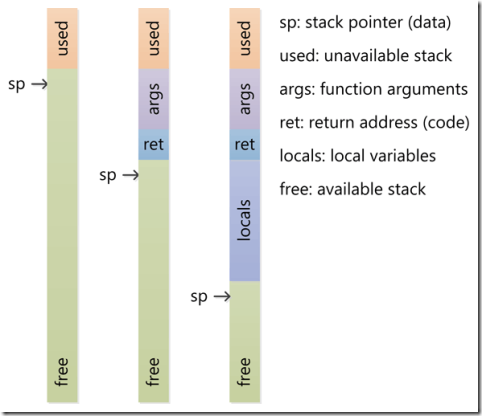4.2 算术表达式的求值

Stack使用的一个最经典的例子就是算术表达式的求值了，这其中还包括前缀表达式和后缀表达式的求值。E. W. Dijkstra发明了使用两个Stack，一个保存操作值，一个保存操作符的方法来实现表达式的求值，具体步骤如下：

1) 当输入的是值的时候Push到属于值的栈中。

2) 当输入的是运算符的时候，Push到运算符的栈中。

3) 当遇到左括号的时候，忽略

4) 当遇到右括号的时候，Pop一个运算符，Pop两个值，然后将计算结果Push到值的栈中。

``````/// <summary>
/// 一个简单的表达式运算
/// </summary>
/// <param name="args"></param>
static void Main(string[] args)
{
Stack<char> operation = new Stack<char>();
Stack<Double> values = new Stack<double>();
//为方便，直接使用ToChar对于两位数的数组问题

foreach (char s in charArray)
{
if (s.Equals('(')) { }
else if (s.Equals('+')) operation.Push(s);
else if (s.Equals('*')) operation.Push(s);
else if (s.Equals(')'))
{
char op = operation.Pop();
if (op.Equals('+'))
values.Push(values.Pop() + values.Pop());
else if (op.Equals('*'))
values.Push(values.Pop() * values.Pop());
}
else values.Push(Double.Parse(s.ToString()));
}
Console.WriteLine(values.Pop());
}``````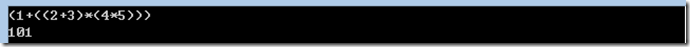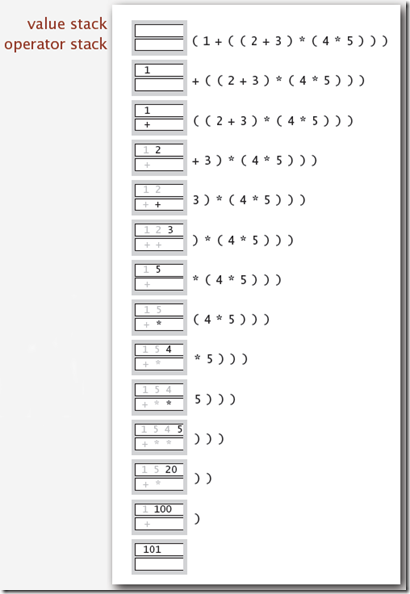4.3 Object-C中以及OpenGL中的图形绘制

``````- (void)drawGreenCircle:(CGContextRef)ctxt {
UIGraphicsPushContext(ctxt);
[[UIColor greenColor] setFill];
// draw my circle
UIGraphicsPopContext();
}

- (void)drawRect:(CGRect)aRect {
CGContextRef context = UIGraphicsGetCurrentContext();
[[UIColor redColor] setFill];
// do some stuff
[self drawGreenCircle:context];
// do more stuff and expect fill color to be red
}``````

4.4 一些其他场景

Queue的应用

# 5.一点点感悟

``````public static void Reverse(int[] array, int begin, int end)
{
while (end > begin)
{
int temp = array[begin];
array[begin] = array[end];
array[end] = temp;

begin++;
end--;
}
}``````

Do you have any suggestions for improvement?